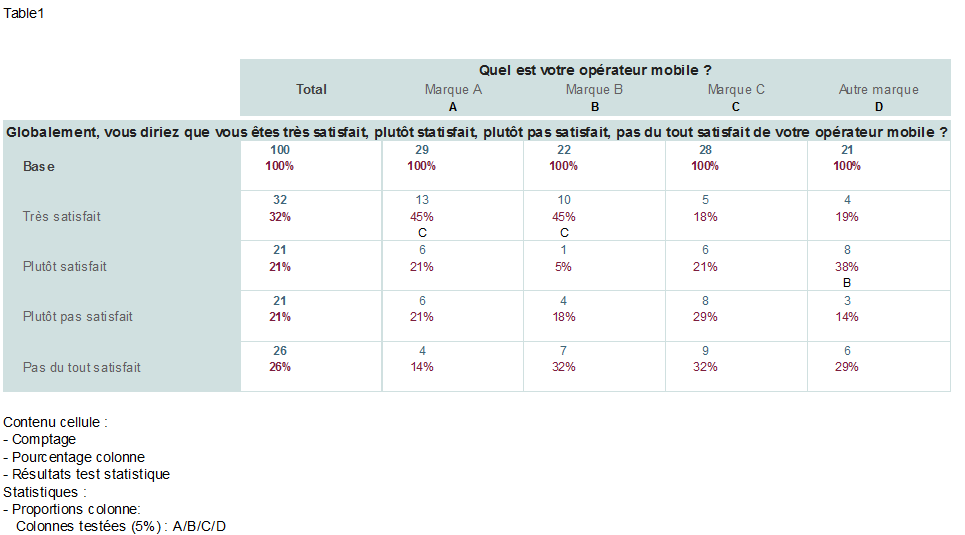15 October 2015

# IBM SPSS Data Collection – Adding statistical tests

Data & Statistics

Statistical tests often need to be performed on survey data to test for differences between various sub-populations.

Today we are going to focus on the “comparison test of column proportions” (“column versus column”), but the same principle also applies to other tests such as the “comparison test of means” for example.
In “Unicom Intelligence (formerly IBM SPSS Data Collection)“, there are multiple ways to specify this test (we will put aside issues to do with the statistical validity of performing multiple tests without prior hypotheses).
One method is to specify the test in the general settings of the MRS file and it will automatically be applied to all tables:

```With TableDoc.Default
With .Statistics

.ColumnProportions.SigLevel = 5

End With
End With
```

(Note: this is to test at the 5% significance level)

The test can also specify it for a particular table. For example:

```With TableDoc.Tables

.Table1.Statistics.ColumnProportions.SigLevel = 5

End With
```

This produces a table like the one below:By default, the statistical test was applied automatically to all columns not of type “base” but tables can be customised. We might not want, for example, to apply the test to the “Other Brand” column (letter D in the example above) and apply it instead to the “Total” column, which is an element of type “base”.

One way to do this, is to specify the table as follows:

```With TableDoc.Tables

.Table1.Statistics.ColumnProportions.SigLevel = 5

.Table1.Statistics.ColumnIDs = "ZABC."
.Table1.Statistics.TestColumns = "Z/A/B/C"

End With
```

(Note: the column IDs are assigned in order with “full-stop” used to specify the column to be excluded from the test)

This produces the following table:But what happens if we want to apply the same rule to all the tables specified in our MRS file? Unfortunately this is not possible! The “ColumnIDs” and “TestColumns” options are not available in the general parameters of the tables. We must therefore add the parameters to the definition of each of the tables, which is a bit cumbersome.

However, there is a useful trick to save some time: a loop can be used on the defined tables to automatically apply the required parameters to “ColumnIDs” and “TestColumns”.

Here’s an example, which can be placed just before filling the tables “Populate ()

```Dim MyTable

For Each MyTable in TableDoc.Tables

MyTable.Statistics.ColumnIDs = "ZABC."
MyTable.Statistics.TestColumns = "Z/A/B/C"

Next
```

Quite a time saver! 😉